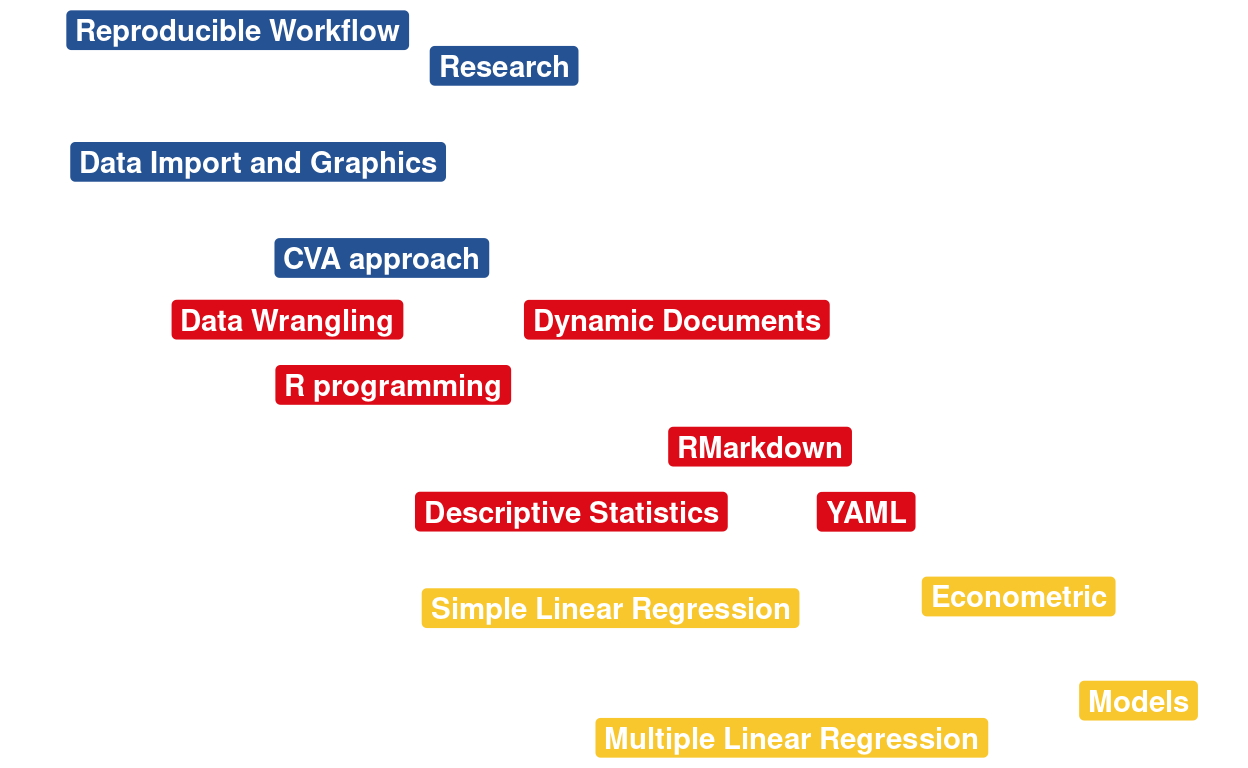# [R Course] R nanocourse 6. Simple Linear Regression

Learn the basic step to produce an econometric analysis with R.

05-23-2019## Introduction

In this session, you will learn the basic step to produce your first econometric analysis with R. Two models will be explained, the simple and the multiple linear regression. This is part of the C-V-A approach, and from now (and the next laboratories) we will explore the -A-, namely the Analysis part of the process.

## Goals

At the end of the session, you should be able to:

1. produce a simple linear regression on a dataset;
2. visualize the relation between two variables;
3. explore a multiple regression.

In the last section of this session, you will find additional materials to go a step further.

### Citation

`Warin (2019, May 23). Thierry Warin, PhD: [R Course] R nanocourse 6. Simple Linear Regression. Retrieved from https://warin.ca/posts/rcourse-rnanocourse6/`
```@misc{warin2019[r,